### Detecting Outliers

You can use the OUTLIER statement to detect changes in the level of the response series that are not accounted for by the estimated model. The types of changes considered are additive outliers (AO), level shifts (LS), and temporary changes (TC).

Let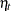be a regression variable that describes some type of change in the mean response. In time series literatureis called a shock signature. An additive outlier at some time pointcorresponds to a shock signaturesuch that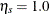andis 0.0 at all other points. Similarly a permanent level shift that originates at timehas a shock signature such thatis 0.0 for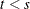and 1.0 for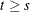. A temporary level shift of duration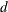that originates at timehasequal to 1.0 betweenand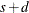and 0.0 otherwise.

Suppose that you are estimating the ARIMA model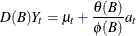where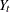is the response series,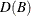is the differencing polynomial in the backward shift operator B (possibly identity),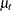is the transfer function input,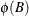and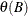are the AR and MA polynomials, respectively, and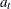is the Gaussian white noise series.

The problem of detection of level shifts in the OUTLIER statement is formulated as a problem of sequential selection of shock signatures that improve the model in the ESTIMATE statement. This is similar to the forward selection process in the stepwise regression procedure. The selection process starts with considering shock signatures of the type specified in the TYPE= option, originating at each nonmissing measurement. This involves testing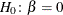versus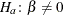in the model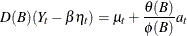for each of these shock signatures. The most significant shock signature, if it also satisfies the significance criterion in ALPHA= option, is included in the model. If no significant shock signature is found, then the outlier detection process stops; otherwise this augmented model, which incorporates the selected shock signature in its transfer function input, becomes the null model for the subsequent selection process. This iterative process stops if at any stage no more significant shock signatures are found or if the number of iterations exceeds the maximum search number that results due to the MAXNUM= and MAXPCT= settings. In all these iterations, the parameters of the ARIMA model in the ESTIMATE statement are held fixed.

The precise details of the testing procedure for a given shock signatureare as follows:

The preceding testing problem is equivalent to testingversusin the following regression with ARMA errors model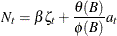where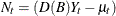is the noise process and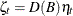is the effective shock signature.

In this setting, under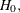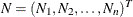is a mean zero Gaussian vector with variance covariance matrix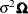. Here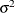is the variance of the white noise processand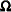is the variance-covariance matrix associated with the ARMA model. Moreover, under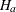,
has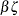as the mean vector where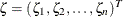. Additionally, the generalized least squares estimate ofand its variance is given by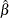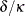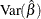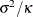where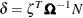and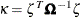. The test statistic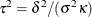is used to test the significance of, which has an approximate chi-squared distribution with 1 degree of freedom under. The type of estimate ofused in the calculation of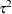can be specified by the SIGMA= option. The default setting is SIGMA=ROBUST, which corresponds to a robust estimate suggested in an outlier detection procedure in X-12-ARIMA, the Census Bureau’s time series analysis program; see Findley et al. (1998) for additional information. The robust estimate ofis computed by the formula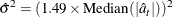where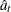are the standardized residuals of the null ARIMA model. The setting SIGMA=MSE corresponds to the usual mean squared error estimate (MSE) computed the same way as in the ESTIMATE statement with the NODF option.

The quantities
and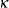are efficiently computed by a method described in de Jong and Penzer (1998); see also Kohn and Ansley (1985).

#### Modeling in the Presence of Outliers

In practice, modeling and forecasting time series data in the presence of outliers is a difficult problem for several reasons. The presence of outliers can adversely affect the model identification and estimation steps. Their presence close to the end of the observation period can have a serious impact on the forecasting performance of the model. In some cases, level shifts are associated with changes in the mechanism that drives the observation process, and separate models might be appropriate to different sections of the data. In view of all these difficulties, diagnostic tools such as outlier detection and residual analysis are essential in any modeling process.

The following modeling strategy, which incorporates level shift detection in the familiar Box-Jenkins modeling methodology, seems to work in many cases:

1. Proceed with model identification and estimation as usual. Suppose this results in a tentative ARIMA model, say M.

2. Check for additive and permanent level shifts unaccounted for by the model M by using the OUTLIER statement. In this step, unless there is evidence to justify it, the number of level shifts searched should be kept small.

3. Augment the original dataset with the regression variables that correspond to the detected outliers.

4. Include the first few of these regression variables in M, and call this model M1. Reestimate all the parameters of M1. It is important not to include too many of these outlier variables in the model in order to avoid the danger of over-fitting.

5. Check the adequacy of M1 by examining the parameter estimates, residual analysis, and outlier detection. Refine it more if necessary.